Linear Algebra & Differential Equations

Sample SageMath Codes for

Linear Algebra & Differential Equations

1. Vector Computations2. Matrix Computations

(1) RREF(2) Determinant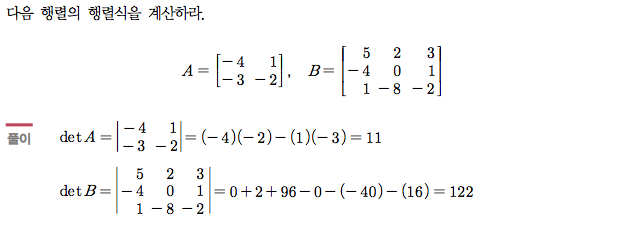(3) Basis and Dimension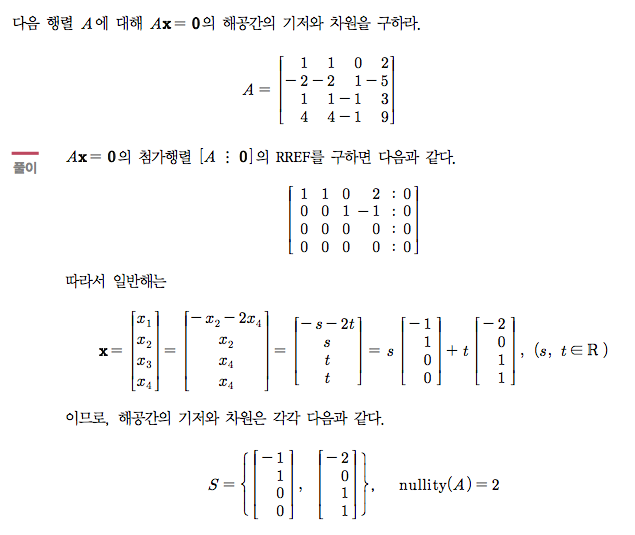(4) Eigensystem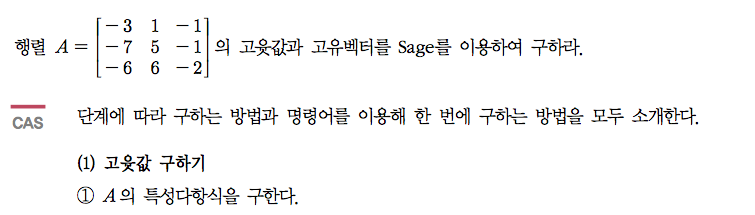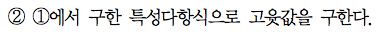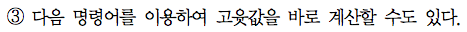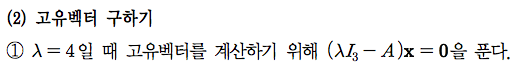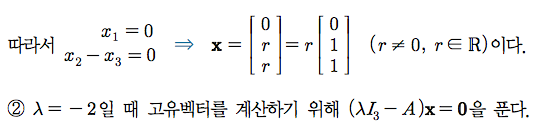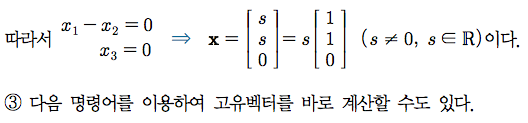3. Differential Equations

(1) 1st order ODE

- initial value problem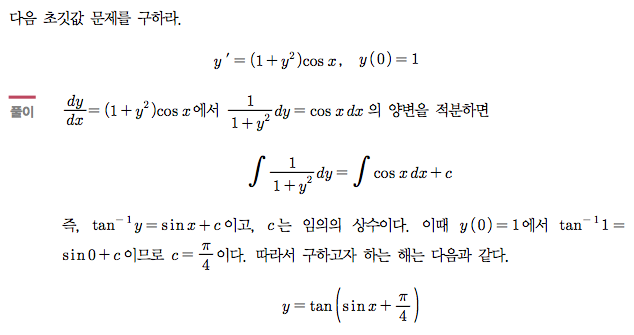- integrating factor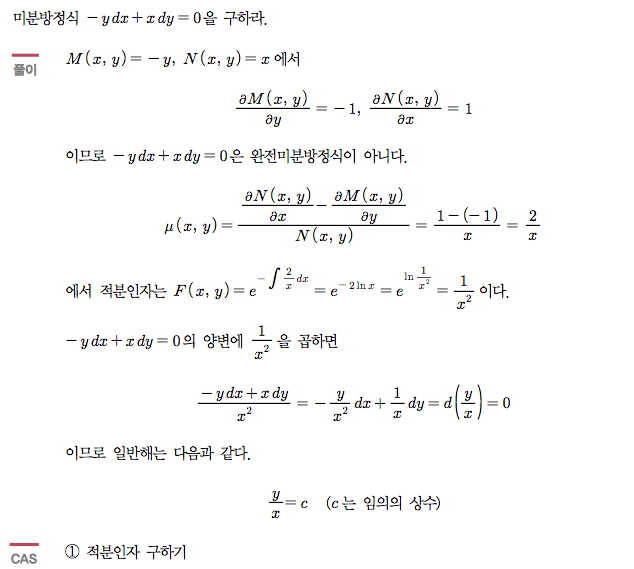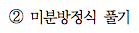(2) 2nd order ODE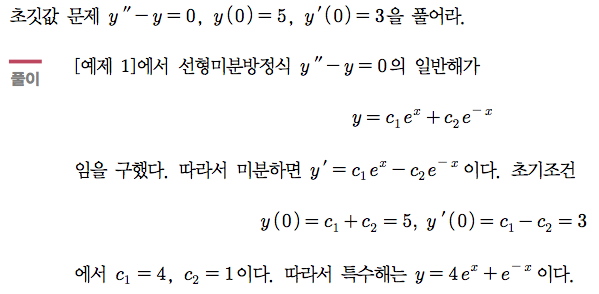(3) Higher order ODE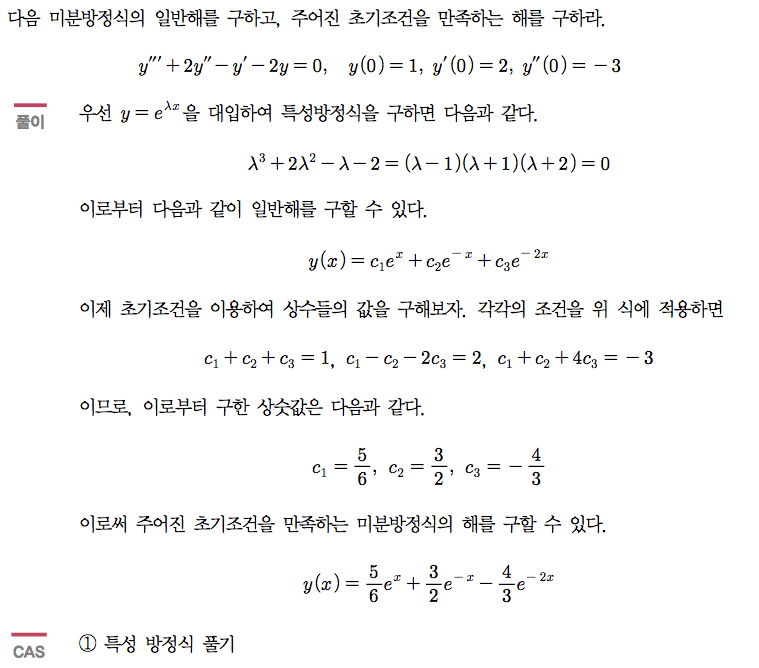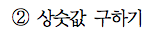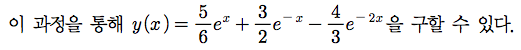(4) Systems of DEs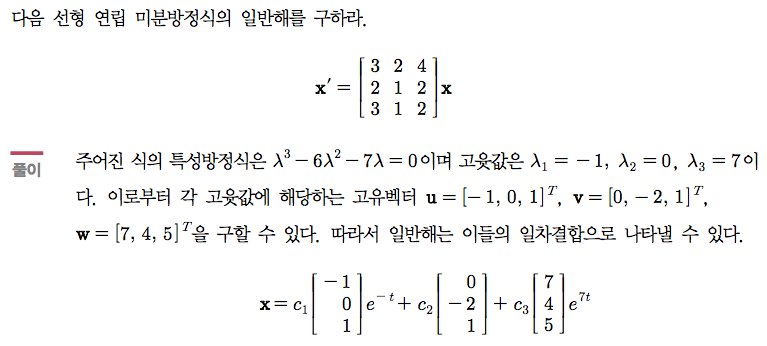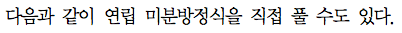(5) Laplace Transform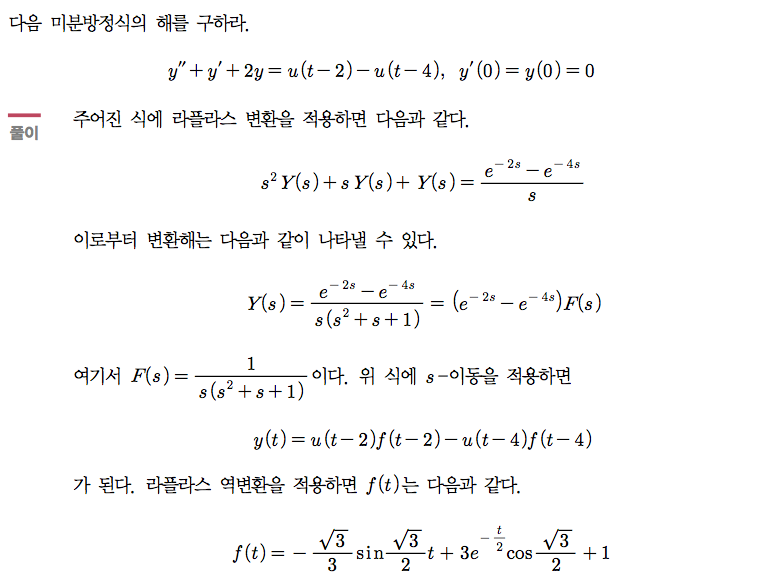(Practice your own SageMath codes in the cells below.)

[References]

1. [Bigbook] Linear Algebra (English version), Sang-Gu Lee et al., Kyobo Books, POD (2016. 1.20)

2. [빅북] 선형대수학 (Korean), 이상구 with 이재화, 김경원, 교보문고 POD (2014. 9. 12)

18. 현대선형대수학 with Sage (Linear Algebra with Sage), 이상구 with 이재화, 김덕선. ISBN 978-89-6105-568-0, 경문사 (2012, 9. 1.).

3. 최신 공학수학 with Sage, 이상구, 김영록, 박준현, 김응기, 이재화, 한빛아카데미 (2016, 1. 12)

[Cyber/Online Laboratories]

[Linear Algebra]

2. (English version) http://matrix.skku.ac.kr/LA/

[Engineering Mathematics]

1. (Korean) http://www.hanbit.co.kr/EM/sage/